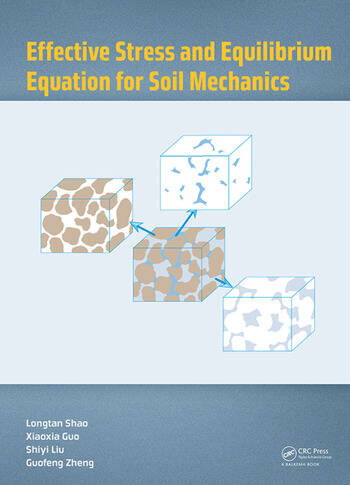# Effective Stress and Equilibrium Equation for Soil Mechanics

## 1st Edition

Longtan Shao, Xiaoxia Guo, Shiyi Liu, Guofeng Zheng

CRC Press
Published December 22, 2017
Reference - 150 Pages
ISBN 9781138092310 - CAT# K34837

For Instructors Request Inspection Copy

USD\$145.00

FREE Standard Shipping!

## Preview

### Summary

The concept of effective stress and the effective stress equation is fundamental for establishing the theory of strength and the relationship of stress and strain in soil mechanics and poromechanics. However, up till now, the physical meaning of effective stress has not been explained clearly, and the theoretical basis of the effective stress equation has not been proposed. Researchers have not yet reached a common understanding of the feasibility of the concept of effective stress and effective stress equation for unsaturated soils.
Effective Stress and Equilibrium Equation for Soil Mechanics discusses the definition of the soil skeleton at first and clarifies that the soil skeleton should include a fraction of pore water. When a free body of soil skeleton is taken to conduct internal force analysis, the stress on the surface of the free body has two parts: one is induced by pore fluid pressure that only includes normal stress; the other is produced by all the other external forces excluding pore fluid pressure. If the effective stress is defined as the soil skeleton stress due to all the external forces excluding pore fluid pressure, the effective stress equation can be easily obtained by the internal force equilibrium analysis. This equation reflects the relationship between the effective stress, total stress and pore fluid pressure, which does not change with the soil property. The effective stress equation of saturated soils and unsaturated soils is unified, i.e., o˜=o˜t –Seuw–(1–Se)ua. For multiphase porous medium, o˜=o˜t –u*,u*=Seiui(i=1,2,...,M). In this book, a theoretical formula of the coefficient of permeability for unsaturated soils is derived. The formula of the seepage force is modified based on the equilibrium differential equation of the pore water. The relationship between the effective stress and the shear strength and deformation of unsaturated soils is preliminarily verified. Finally, some possibly controversial problems are discussed to provide a better understanding of the role of the equilibrium equation and the concept of effective stress.

#### Instructors

We provide complimentary e-inspection copies of primary textbooks to instructors considering our books for course adoption.